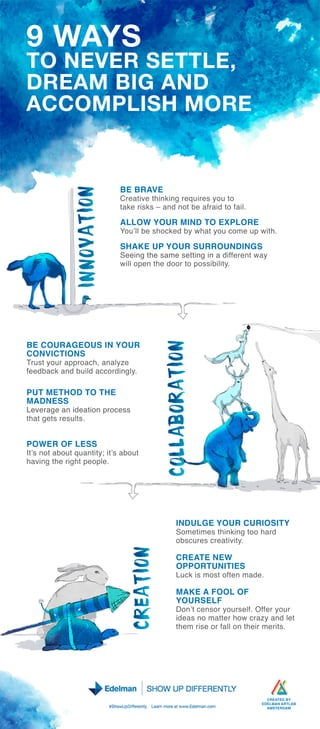Successfully reported this slideshow.×
1 of 1

9 Ways to Show Up Differently

151

Share

Defying the status quo takes courage, yet reaps great rewards. “Show Up Differently” are three words that epitomize and humanize the spirit of Edelman and how we act on behalf our clients. We have more than 60 years of firsts, innovations and trailblazing campaigns that have helped our clients succeed.

We won’t stop now. Show Up Differently is an invitation to our industry to join us. All of us can be the architects of a bold new future by showing up differently. Doing so is invigorating and combines passion, smarts, forward thinking and dare we say, a bit of fun.

Here are just nine ways we think about how to show up differently. Join the conversation and tell us how you Show Up Differently.

http://www.edelman.com/show-up-differently/

More Related Content

See all

See all

9 Ways to Show Up Differently

1. 1. 9WA S Y B B AE E R V C e t etik grq i sy ut rai hn i e ur o o v n e tk r k –a dn t eari t fi a e i s n o b f d o al s a . AL L OW Y R MI D T E P OR OU N O XL E Y ul es o k db w a y uc meu wt. o ’b h ce y h t o o l p i h S A EU Y R S R OU D N H K P OU U R N I GS S en tes mes tn i adf rn w y e ig h a et g n iee t a i f wl p nted o t p s ii y i o e h o ro o s l . l bi t B C R GE SI Y R E OU A OU N OU C VC I S ON I TON Tu t o r p ra h a ay e rs y u a po c , n l z fe b c a db i a c rigy e d a k n ul c odn l d . P TME H U T OD T T E O H MA N S D ES L v rg a ie t npo e s e ea e n d ai rc s o ta g t rs l . h t es e ut s P OWE OFL S R ES Isn t b u q a ty i a o t t o a o t u ni ;t b u ’ t ’ s h v gter h p o l. a i h i t e pe n g OL B R TO C LA O A IN N O A IN IN V TO T N V RS T L , O EE ETE D E M BG A D R A I N A C C OMP IH MOR LS E R A IN C E TO I D L Y R C R OST N U GE OU U I IY S mei stik gtoh r o t me hn i o ad n o s ue ce t i. b c rs rait vy C ET N W R AE E OP OR U IIS P T N TE L c i mo t f nma e u k s s ot e d. MA EAF K OOLOF Y R EF OU S L D nt e s r o refOf r o r o ’c n o y us l f y u . e ie sn matr o ca ya dlt d a o t h w rz n e e te r eo fl nter rs h m i ral h imei . s o t C E T DB R AE Y E E MA A T A D L N RLB A TR A MS E D M